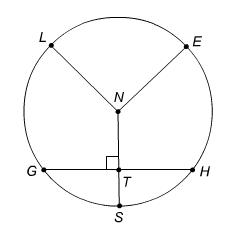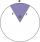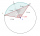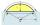# Chord - TS

The radius of circle k measures 68 cm. Arc GH = 47 cm. What is TS?

|TS| =  4.02 cm

### Step-by-step explanation:Did you find an error or inaccuracy? Feel free to write us. Thank you!Tips to related online calculators

#### You need to know the following knowledge to solve this word math problem:

We encourage you to watch this tutorial video on this math problem:

## Related math problems and questions:

• Chord - TS v2The radius of circle k measures 87 cm. Chord GH = 22 cm. What is TS?
• Chord MNChord MN of circle has distance from the center circle S 120 cm. Angle MSN is 64°. Determine the radius of the circle.
• Circular sectorI have a circular sector with a length 15 cm with an unknown central angle. It is inscribed by a circle with radius 5 cm. What is the central angle alpha in the circular sector?
• Arc and segmentCalculate the length of circular arc l, area of the circular arc S1 and area of circular segment S2. Radius of the circle is 11 and corresponding angle is (2)/(12) π.
• Arc-sectorarc length = 17 cm area of sector = 55 cm2 arc angle = ? the radius of the sector = ?
• SectorThe perimeter of a circular sector with an angle 1.8 rad is 64 cm. Determine the radius of the circle from which the sector comes.
• Length of the chordCalculate the length of the chord in a circle with a radius of 25 cm with a central angle of 26°.
• Regular n-gonWhich regular polygon have a radius of circumscribed circle r = 10 cm and the radius of inscribed circle p = 9.962 cm?
• Circle sectorThe circular sector with a central angle 160° has an area 452 cm2. Calculate its radius r.
• Equilateral triangleHow long should be the minimum radius of the circular plate to be cut equilateral triangle with side 19 cm from it?
• Circle arcCalculate the circular arc area in m2 where the diameter is 263 dm, and a central angle is 40°. Result round to three decimal places.
• TriangleCalculate the area of ​​the triangle ABC if b = c = 17 cm, R = 19 cm (R is the circumradius).
• Hexagon 5The distance of parallel sides of regular hexagonal is 61 cm. Calculate the length of the radius of the circle described to this hexagon.
• n-gonWhat is the side length of the regular 5-gon inscribed in a circle of radius 12 cm?
• IS triangleCalculate interior angles of the isosceles triangle with base 40 cm and legs 22 cm long.
• Right triangle trigonometricsCalculate the size of the remaining sides and angles of a right triangle ABC if it is given: b = 10 cm; c = 20 cm; angle alpha = 60° and the angle beta = 30° (use the Pythagorean theorem and functions sine, cosine, tangent, cotangent)
• Height of the arc - formulaCalculate the height of the arc if the length of the arc is 77 and chord length 40. Does exist a formula to solve this?